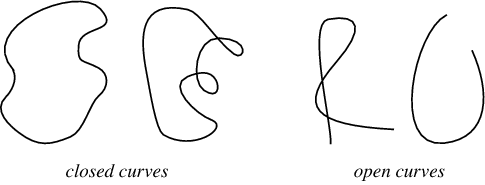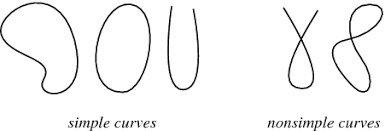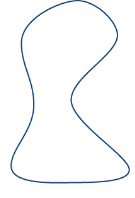# Explain the types of curves with appropriate diagrams.

Solution :

We have to explain the types of curves with appropriate diagrams.

Closed curve:

A closed curve should not have end points.Simple curves:

Simple curves do not cross or intersectSimple closed curve:

Does not have end points and does not intersectPolygon is a simple closed curve, but it should be made of line segments.

Example

triangle, square etc.

To draw curves we need to know the shape that we have to draw.

Updated on: 10-Oct-2022

59 Views